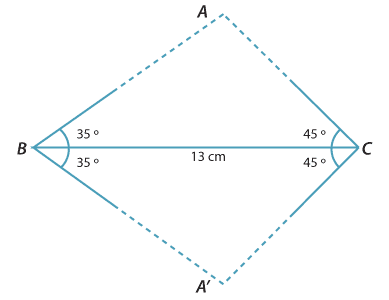#### Constructing a triangle with two angles and a given side

When the angles of a triangle and one side are known, however, there is no longer any freedom for the size to change, so that only one such triangle can be constructed (up to congruence). To demonstrate this, suppose that we are asked to construct a triangle ABC with these angles and side length:

$$B=35\,^{\circ}$$$$C=45\,^{\circ}$$$$BC = 13\,\text{cm}$$(It follows that $$A=100\,^{\circ}$$.)

The most straightforward way is to draw the interval BC and then construct the angles at the endpoints B and C.

Triangle A′BC is the reflection of triangle ABC in BC.Detailed description

Any triangle with angles $$35\,^{\circ}$$ and $$45\,^{\circ}$$ and a side of length 13 cm 'in between' these two angles is congruent to triangle $$ABC$$.